# logistic和softmax原理、联系

logistic原文：http://blog.csdn.net/ariessurfer/article/details/41310525

softmax原文：http://blog.csdn.net/pi9nc/article/details/19336629

Logistic回归为概率型非线性回归模型，是研究二分类观察结果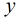与一些影响因素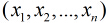之间关系的一种多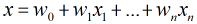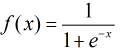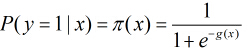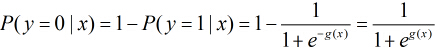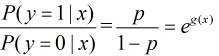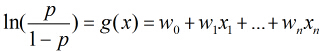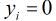的概率为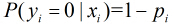，所以得到一个观测值的概率为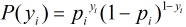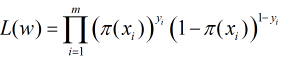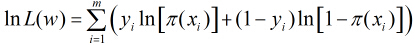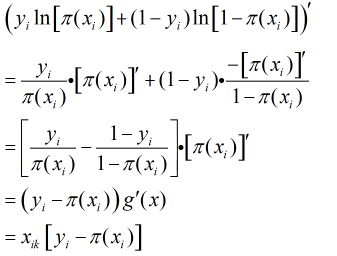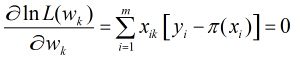# Softmax回归

[hide]

## 简介\begin{align}h_\theta(x) = \frac{1}{1+\exp(-\theta^Tx)},\end{align}\begin{align}J(\theta) = -\frac{1}{m} \left[ \sum_{i=1}^m y^{(i)} \log h_\theta(x^{(i)}) + (1-y^{(i)}) \log (1-h_\theta(x^{(i)})) \right]\end{align}

（求和符号要放在中括号外面）在 softmax回归中，我们解决的是多分类问题（相对于 logistic 回归解决的二分类问题），类标$\textstyle y$ 可以取$\textstyle k$ 个不同的值（而不是 2 个）。因此，对于训练集$\{ (x^{(1)}, y^{(1)}), \ldots, (x^{(m)}, y^{(m)}) \}$，我们有$y^{(i)} \in \{1, 2, \ldots, k\}$。（注意此处的类别下标从 1 开始，而不是 0）。例如，在 MNIST 数字识别任务中，我们有$\textstyle k=10$ 个不同的类别。\begin{align}h_\theta(x^{(i)}) =\begin{bmatrix}p(y^{(i)} = 1 | x^{(i)}; \theta) \\p(y^{(i)} = 2 | x^{(i)}; \theta) \\\vdots \\p(y^{(i)} = k | x^{(i)}; \theta)\end{bmatrix}=\frac{1}{ \sum_{j=1}^{k}{e^{ \theta_j^T x^{(i)} }} }\begin{bmatrix}e^{ \theta_1^T x^{(i)} } \\e^{ \theta_2^T x^{(i)} } \\\vdots \\e^{ \theta_k^T x^{(i)} } \\\end{bmatrix}\end{align}$\theta = \begin{bmatrix}\mbox{---} \theta_1^T \mbox{---} \\\mbox{---} \theta_2^T \mbox{---} \\\vdots \\\mbox{---} \theta_k^T \mbox{---} \\\end{bmatrix}$

## 代价函数$\textstyle 1\{​$ 值为真的表达式$\textstyle \}=1$


，$\textstyle 1\{​$ 值为假的表达式$\textstyle \}=0$。举例来说，表达式$\textstyle 1\{2+2=4\}$ 的值为1 ，$\textstyle 1\{1+1=5\}$的值为 0。我们的代价函数为：\begin{align}J(\theta) = - \frac{1}{m} \left[ \sum_{i=1}^{m} \sum_{j=1}^{k} 1\left\{y^{(i)} = j\right\} \log \frac{e^{\theta_j^T x^{(i)}}}{\sum_{l=1}^k e^{ \theta_l^T x^{(i)} }}\right]\end{align}\begin{align}J(\theta) &= -\frac{1}{m} \left[ \sum_{i=1}^m (1-y^{(i)}) \log (1-h_\theta(x^{(i)})) + y^{(i)} \log h_\theta(x^{(i)}) \right] \\&= - \frac{1}{m} \left[ \sum_{i=1}^{m} \sum_{j=0}^{1} 1\left\{y^{(i)} = j\right\} \log p(y^{(i)} = j | x^{(i)} ; \theta) \right]\end{align}$p(y^{(i)} = j | x^{(i)} ; \theta) = \frac{e^{\theta_j^T x^{(i)}}}{\sum_{l=1}^k e^{ \theta_l^T x^{(i)}} }$.\begin{align}\nabla_{\theta_j} J(\theta) = - \frac{1}{m} \sum_{i=1}^{m}{ \left[ x^{(i)} \left( 1\{ y^{(i)} = j\} - p(y^{(i)} = j | x^{(i)}; \theta) \right) \right] }\end{align}

## Softmax回归模型参数化的特点

Softmax 回归有一个不寻常的特点：它有一个“冗余”的参数集。为了便于阐述这一特点，假设我们从参数向量$\textstyle \theta_j$ 中减去了向量$\textstyle \psi$，这时，每一个$\textstyle \theta_j$ 都变成了$\textstyle \theta_j - \psi$($\textstyle j=1, \ldots, k$)。此时假设函数变成了以下的式子：\begin{align}p(y^{(i)} = j | x^{(i)} ; \theta)&= \frac{e^{(\theta_j-\psi)^T x^{(i)}}}{\sum_{l=1}^k e^{ (\theta_l-\psi)^T x^{(i)}}} \\&= \frac{e^{\theta_j^T x^{(i)}} e^{-\psi^Tx^{(i)}}}{\sum_{l=1}^k e^{\theta_l^T x^{(i)}} e^{-\psi^Tx^{(i)}}} \\&= \frac{e^{\theta_j^T x^{(i)}}}{\sum_{l=1}^k e^{ \theta_l^T x^{(i)}}}.\end{align}

## 权重衰减\begin{align}J(\theta) = - \frac{1}{m} \left[ \sum_{i=1}^{m} \sum_{j=1}^{k} 1\left\{y^{(i)} = j\right\} \log \frac{e^{\theta_j^T x^{(i)}}}{\sum_{l=1}^k e^{ \theta_l^T x^{(i)} }} \right] + \frac{\lambda}{2} \sum_{i=1}^k \sum_{j=0}^n \theta_{ij}^2\end{align}\begin{align}\nabla_{\theta_j} J(\theta) = - \frac{1}{m} \sum_{i=1}^{m}{ \left[ x^{(i)} ( 1\{ y^{(i)} = j\} - p(y^{(i)} = j | x^{(i)}; \theta) ) \right] } + \lambda \theta_j\end{align}

## Softmax回归与Logistic 回归的关系\begin{align}h_\theta(x) &=\frac{1}{ e^{\theta_1^Tx} + e^{ \theta_2^T x^{(i)} } }\begin{bmatrix}e^{ \theta_1^T x } \\e^{ \theta_2^T x }\end{bmatrix}\end{align}\begin{align}h(x) &=\frac{1}{ e^{\vec{0}^Tx} + e^{ (\theta_2-\theta_1)^T x^{(i)} } }\begin{bmatrix}e^{ \vec{0}^T x } \\e^{ (\theta_2-\theta_1)^T x }\end{bmatrix} \\&=\begin{bmatrix}\frac{1}{ 1 + e^{ (\theta_2-\theta_1)^T x^{(i)} } } \\\frac{e^{ (\theta_2-\theta_1)^T x }}{ 1 + e^{ (\theta_2-\theta_1)^T x^{(i)} } }\end{bmatrix} \\&=\begin{bmatrix}\frac{1}{ 1 + e^{ (\theta_2-\theta_1)^T x^{(i)} } } \\1 - \frac{1}{ 1 + e^{ (\theta_2-\theta_1)^T x^{(i)} } } \\\end{bmatrix}\end{align}

## 中英文对照

Softmax回归 Softmax Regression

logistic回归 logistic regression

## 中文译者

04-039973
01-01

10-261021
04-16423
09-11471
11-2827万+
10-177907
09-0255万+
07-151760
11-06104
05-303618
07-17156
10-214986
05-051056
07-032515
12-20697
06-27477
01-08875
06-143675
03-091206

### “相关推荐”对你有帮助么？

•非常没帮助
•没帮助
•一般
•有帮助
•非常有帮助点击重新获取扫码支付余额充值